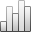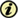Acta Stereologica

0351-580XMore Statisticssince 05 December 2013 :
View(s): 179 (1 ULiège)
Jan Rataj

### Estimation of oriented normal direction distribution via mixed areas

Article#### Abstract

An indirect method for the estimation of the area measure of a planar set X from the convex ring is proposed. At first, the mixed area A(X,ﬠ,K) of X with sufficiently enough rotations ﬠ of a chosen convex test set K is estimated by means of the dilation area. Then, the area measure estimate is obtained by solving an integral equation. The estimation bias is discussed and several particular examples are presented.

Keywords : direction distribution, unit outer normal, convex ring, dilation area, mixed area, area measure, estimation variance